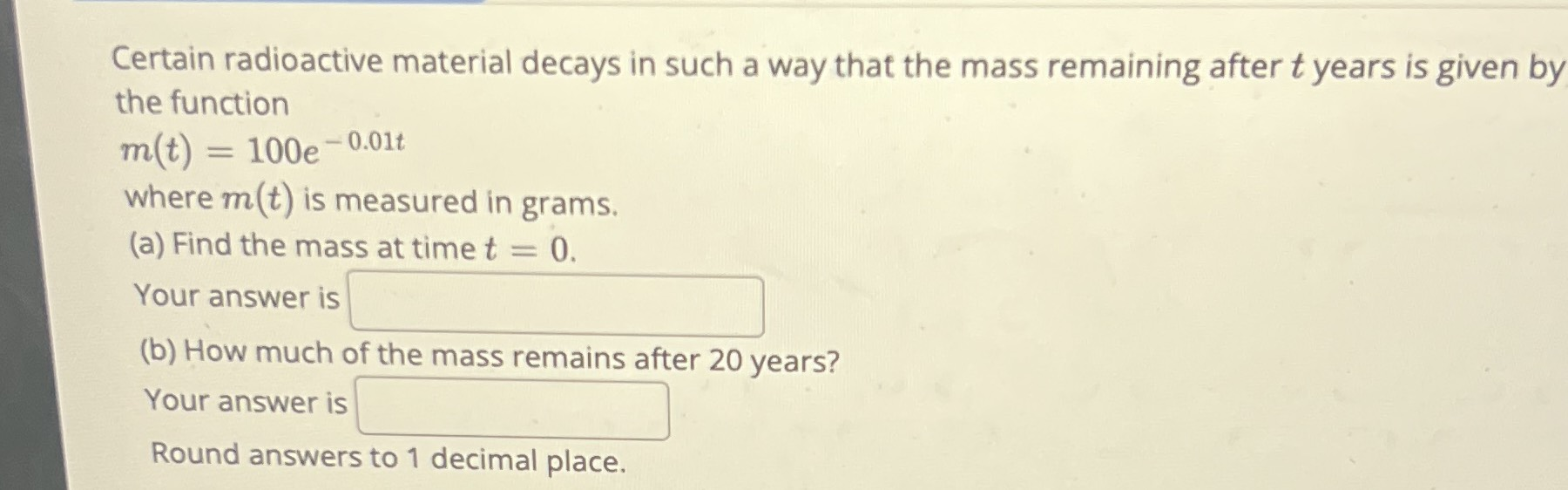### ¿Todavía tienes preguntas de matemáticas?

Pregunte a nuestros tutores expertos
Algebra
PreguntaCertain radioactive material decays in such a way that the mass remaining after $$t$$ years is given by the function

$$m ( t ) = 100 e ^ { - 0.01 t }$$

where $$m ( t )$$ is measured in grams. (a) Find the mass at time $$t = 0$$ . Your answer is (b) How much of the mass remains after $$20$$ years? Your answer is Round answers to $$1$$ decimal place.